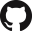# 30.003 Introduction to Probability and Statistics¶

No. of Credits: 12 Subject Credits

Pre-requisites:

## Goal¶

The aim of this course is to introduce concepts of Probability and Statistics.

## Learning Objectives¶

• Apply key concepts of probability, including discrete and continuous random variables, probability distributions, conditioning, independence, expectations, and moments
• Define and explain the different statistical distributions (e.g., normal, log-normal, Poisson) and the typical phenomena that each distribution often describes
• Apply the basic rules and theorems in probability including Bayes’s theorem and the Central Limit Theorem (CLT)
• Define and demonstrate the concepts of estimation and properties of estimators
• Apply the concepts of interval estimation and confidence intervals
• Apply the concepts of hypothesis testing and p-value
• Apply the method of least squares to estimate the parameters in a regression model
• Use software to facilitate statistical analysis

## Measurable Outcomes¶

• Evaluate the probabilities and conditional probabilities
• Evaluate expectations and conditional expectations of random variables
• Approximate the distribution of sum of random variables using CLT
• Construct point estimators using the method of maximum likelihood
• Calculate the number of samples needed to construct confidence levels on the mean and variance of a normal distribution
• Design hypothesis tests for a given set of data and select the appropriate thresholds for the tests
• Use linear regression analysis to develop an empirical model of experimental data.
• Apply computer programs to facilitate the analysis of data.

## Pedagogy¶

• Cohort based lecture
• Hands-on projects

## Text & References¶

• Class participation: 5%
• Homework: 25%
• Project: 10%
• Mid-term exam: 30%
• Final exam: 30%

## Policies¶

Homework is assigned on every week and is due in the 1st class of the following week.

## Course Notes¶

This set of course notes was graciously shared by Wei Min Cher, updated as of 05 January 2020.